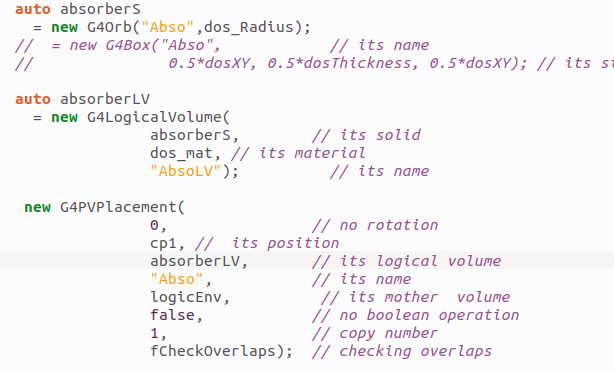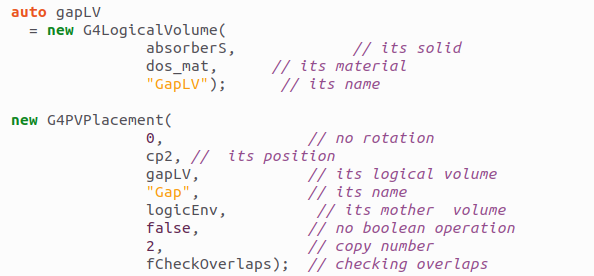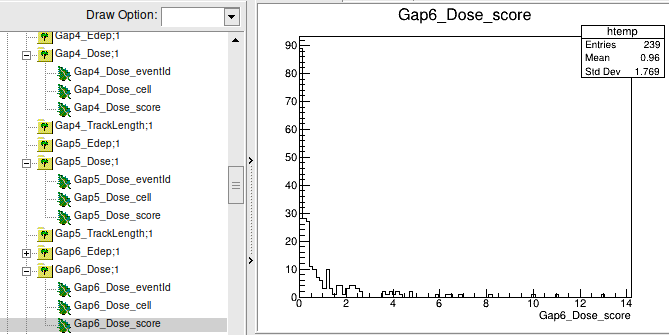# Am I calculating the absorbed dose correctly?

Hello everyone, my question is, am I reading the absorbed dose correctly? I am using the B4d example for my purposes. Source of neutrons with an energy of 14.1 MeV. I am using a spherical detector made of G4_MUSCLE_WITH_SUCROSE material.
After calculations in the root file, I use Entries and Mean and insert them into the formula in excel (absorbedDose [Sv/h]=Entries *Mean *(1,60Е-13) *3600 *1/72kg (weight of detector). Where 1 ,60E-13 joule = 1MeV. And why do Edep sroce and Dose sroce repeat each other?To get the equivalent dose, I multiply the absorbed by the weighting factor. How to derive the energy spectrum to better determine the coefficient? I am attaching the code.
T.y for help)